# JP2016138870A - Semiconductor device - Google Patents

## Info

Publication number
JP2016138870A
JP2016138870A JP2015015618A JP2015015618A JP2016138870A JP 2016138870 A JP2016138870 A JP 2016138870A JP 2015015618 A JP2015015618 A JP 2015015618A JP 2015015618 A JP2015015618 A JP 2015015618A JP 2016138870 A JP2016138870 A JP 2016138870A
Authority
JP
Japan
Prior art keywords
signal
input
angle
switch circuit
switch
Prior art date
Legal status (The legal status is an assumption and is not a legal conclusion. Google has not performed a legal analysis and makes no representation as to the accuracy of the status listed.)
Granted
Application number
JP2015015618A
Other languages
Japanese (ja)
Other versions
JP6527704B2 (en
Inventor

Kazuaki Kurooka

Korehiro Funato

Original Assignee
ルネサスエレクトロニクス株式会社
Renesas Electronics Corp
Priority date (The priority date is an assumption and is not a legal conclusion. Google has not performed a legal analysis and makes no representation as to the accuracy of the date listed.)
Filing date
Publication date
Application filed by ルネサスエレクトロニクス株式会社, Renesas Electronics Corp filed Critical ルネサスエレクトロニクス株式会社
Priority to JP2015015618A priority Critical patent/JP6527704B2/en
Publication of JP2016138870A publication Critical patent/JP2016138870A/en
Application granted granted Critical
Publication of JP6527704B2 publication Critical patent/JP6527704B2/en
Active legal-status Critical Current
Anticipated expiration legal-status Critical

• 239000004065 semiconductors Substances 0.000 title claims abstract description 22
• 230000005284 excitation Effects 0.000 claims description 40
• 230000001360 synchronised Effects 0.000 claims description 13
• 230000001276 controlling effects Effects 0.000 claims description 5
• 230000000051 modifying Effects 0.000 claims description 5
• 230000000694 effects Effects 0.000 description 9
• 238000006243 chemical reactions Methods 0.000 description 5
• 238000000034 methods Methods 0.000 description 1

## Images

•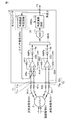•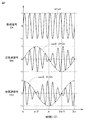•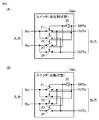•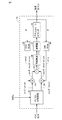•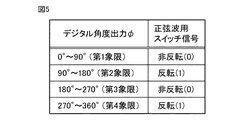•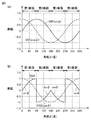•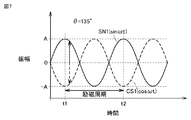•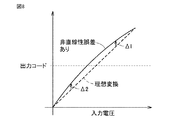•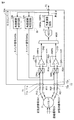•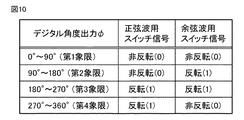•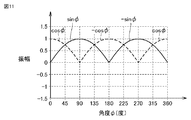•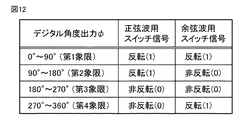•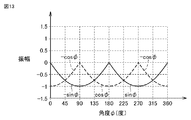•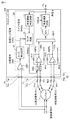•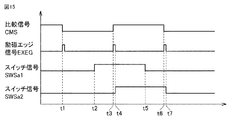•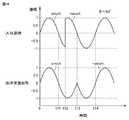•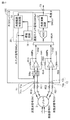•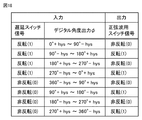•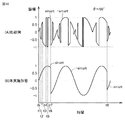•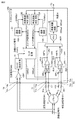•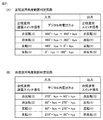•## Classifications

• HELECTRICITY
• H03BASIC ELECTRONIC CIRCUITRY
• H03MCODING; DECODING; CODE CONVERSION IN GENERAL
• H03M1/00Analogue/digital conversion; Digital/analogue conversion
• H03M1/12Analogue/digital converters
• H03M1/48Servo-type converters
• H03M1/485Servo-type converters for position encoding, e.g. using resolvers or synchros
• GPHYSICS
• G01MEASURING; TESTING
• G01BMEASURING LENGTH, THICKNESS OR SIMILAR LINEAR DIMENSIONS; MEASURING ANGLES; MEASURING AREAS; MEASURING IRREGULARITIES OF SURFACES OR CONTOURS
• G01B7/00Measuring arrangements characterised by the use of electric or magnetic means
• G01B7/30Measuring arrangements characterised by the use of electric or magnetic means for measuring angles or tapers; for testing the alignment of axes
• HELECTRICITY
• H03BASIC ELECTRONIC CIRCUITRY
• H03MCODING; DECODING; CODE CONVERSION IN GENERAL
• H03M1/00Analogue/digital conversion; Digital/analogue conversion
• H03M1/06Continuously compensating for, or preventing, undesired influence of physical parameters
• H03M1/08Continuously compensating for, or preventing, undesired influence of physical parameters of noise
• H03M1/0863Continuously compensating for, or preventing, undesired influence of physical parameters of noise of switching transients, e.g. glitches
• H03M1/0872Continuously compensating for, or preventing, undesired influence of physical parameters of noise of switching transients, e.g. glitches by disabling changes in the output during the transitions, e.g. by holding or latching
• HELECTRICITY
• H03BASIC ELECTRONIC CIRCUITRY
• H03MCODING; DECODING; CODE CONVERSION IN GENERAL
• H03M1/00Analogue/digital conversion; Digital/analogue conversion
• H03M1/12Analogue/digital converters
• H03M1/64Analogue/digital converters with intermediate conversion to phase of sinusoidal or similar periodical signals
• H03M1/645Analogue/digital converters with intermediate conversion to phase of sinusoidal or similar periodical signals for position encoding, e.g. using resolvers or synchros

## Abstract

PROBLEM TO BE SOLVED: To suppress an angle detection error resulting from a non-linearity error in an A/D converter.SOLUTION: In a semiconductor device, a sine wave signal SN1 is input to a first input unit T1 and a cosine wave signal CS1 is input to a second input unit T2. A multiplexer MUX alternately selects one of the sine wave signal SN1 and the cosine wave signal CS1. An analog/digital converter 11 converts an output signal from the multiplexer MUX to a digital value. A switch circuit SWa is connected between at least one of the first and second input units T1 and T2 and the multiplexer MUX. A switch circuit SWSa is configured to be able to invert the input sine wave signal SN1 or cosine wave signal CS1.SELECTED DRAWING: Figure 1

## Description

The present invention relates to a semiconductor device, and is used, for example, in a resolver / digital converter that converts a signal output from a resolver into a digital signal.

A resolver is known as a rotation angle sensor used in harsh environments such as for industrial machines or for motors of automobile power systems. The resolver outputs an analog sine wave signal representing a sine of an electrical angle and an analog cosine wave signal representing a cosine of an electrical angle. A resolver / digital converter (hereinafter referred to as an R / D converter) converts the above sine wave signal and cosine wave signal into digital values, and is based on the digitally converted sine wave signal and cosine wave signal. Thus, the electrical angle is calculated (see, for example, Japanese Patent Application Laid-Open No. 2004-309285 (Patent Document 1)).

JP 2004-309285 A

In the R / D converter, normally, a sine wave signal and a cosine wave signal are alternately selected by a multiplexer, and the selected signal is converted into a digital value by a common analog / digital converter (A / D converter). In this case, an error may occur in the finally detected electrical angle due to the non-linearity error of the A / D (Analog to Digital) converter. Such an error is not limited to the R / D converter, and may be a problem that may occur when a plurality of signals are A / D converted in order.

Other problems and novel features will become apparent from the description of the specification and the accompanying drawings.

In the semiconductor device according to the embodiment, a switch circuit for inverting at least one of the sine wave signal and the cosine wave signal is provided in the front stage of the multiplexer.

According to the above embodiment, it is possible to suppress the angle detection error due to the non-linearity error of the A / D converter.

1 is a block diagram showing a configuration of an R / D converter 10 as a semiconductor device according to a first embodiment. It is a figure which shows an example of the excitation signal EX input into the resolver 3 of FIG. 1, and the sine wave signal SN1 and cosine wave signal CS1 output from the resolver 3. FIG. 2 is a circuit diagram illustrating an example of a configuration and operation of a switch circuit SWa in FIG. 1. It is a block diagram which shows an example of a structure of the angle calculation circuit 12 of FIG. It is a figure which shows the relationship between the input value of the angle range determination circuit 13 of FIG. It is a graph which shows the relationship between the amplitude of sine wave signal SN1 and cosine wave signal CS1, and angle (phi). It is a wave form diagram of sine wave signal SN1 and cosine wave signal CS1 when electrical angle θ is 135 °. It is a figure for demonstrating the nonlinearity error of an A / D converter. It is a block diagram which shows the structure of 10 A of R / D converters by 2nd Embodiment. It is a figure which shows the relationship between the input value and output value of angle range determination circuit 13A, 13B of FIG. 9 in a table format. 10 is a graph showing the relationship between the amplitude and the angle φ of the sine wave signal SN2 and cosine wave signal CS2 input to the A / D converter 11 in the R / D converter 10A of FIG. FIG. 10 is a diagram showing a relationship between input values and output values in a tabular form in a modification of the angle range determination circuits 13A and 13B of FIG. In the modification of 2nd Embodiment, it is a graph which shows the relationship between the amplitude of the sine wave signal SN2 and cosine wave signal CS2 which are input into the A / D converter 11, and angle (phi). It is a block diagram which shows the structure of R / D converter 10B by 3rd Embodiment. FIG. 15 is a timing chart showing operation waveforms of respective units in FIG. 14. It is a figure which shows an example of the waveform of the sine wave signal SN2 after the output of amplifier AMPa of FIG. It is a block diagram which shows the structure of 10 C of R / D converters by 4th Embodiment. It is a figure which shows the relationship between the input value of the angle range determination circuit 13 of FIG. It is a figure which shows an example of the waveform of the sine wave signal SN2 after the output of amplifier AMPa of FIG. It is a block diagram which shows the structure of R / D converter 10D by 5th Embodiment. It is a figure which shows the relationship between the input value and output value of angle range determination circuit 13A, 13B of FIG. It is a block diagram which shows the structure of a motor control apparatus.

Hereinafter, each embodiment will be described in detail with reference to the drawings. The same or corresponding parts are denoted by the same reference numerals, and description thereof will not be repeated.

<First Embodiment>
[Configuration of R / D converter]
FIG. 1 is a block diagram showing a configuration of an R / D converter 10 as the semiconductor device according to the first embodiment. In the first embodiment, an R / D converter will be described as an example of the semiconductor device. However, as described in the sixth embodiment, an integrated circuit (for example, a microcontroller) including the R / D converter is described. A semiconductor device can also be configured by a unit.

Referring to FIG. 1, R / D converter 10 includes input units T1 and T2, an output unit T3, amplifiers AMPa and AMPb, resistance elements R13, R14, R23 and R24, a switch circuit SWa, and a multiplexer MUX. And an A / D converter 11, an angle calculation circuit 12, and an angle range determination circuit 13. The R / D converter 10 calculates the angle φ based on the sine wave signal SN1 and the cosine wave signal CS1 received from the resolver 3. Hereinafter, the configuration of each part of the resolver 3 and the R / D converter 10 will be described.

(Resolver)
The resolver 3 includes one rotor winding and two stator windings (not shown). When the shaft angle multiplier is 1, the two stator windings are arranged, for example, by mechanically shifting an angle of 90 °. When the excitation signal EX is inputted to the rotor winding, the sine wave signal SN1 in which the excitation signal EX is modulated by the sine of the electrical angle and the cosine of the electrical angle (cosine: cosine) from the two stator windings. ), The cosine wave signal CS1 in which the excitation signal EX is modulated is output. When the mechanical angle of the rotor is Θ, the electrical angle is θ, and the shaft angle multiplier is N, there is a relationship of θ = N × Θ.

FIG. 2 is a diagram illustrating an example of the excitation signal EX input to the resolver 3 in FIG. 1 and the sine wave signal SN1 and the cosine wave signal CS1 output from the resolver 3. In FIG. 2, it is assumed that the excitation signal EX is sin (ωt) (where ω is an angular frequency and t is time), and the rotor is rotating at an angular velocity Ω (where ω = 10 × Ω). The shaft angle multiplier is 1. In this case, the rotation angle (electrical angle) θ of the rotor has a relationship of θ = Ω · t. Note that the time on the horizontal axis in FIG. 2 is normalized by Ω. In this case, the sine wave signal SN1 is represented by sinωt · sinθ = sinωt · sinΩt, and the cosine wave signal CS1 is represented by sinωt · cosθ = cosωt · cosΩt.

Referring to FIG. 1 again, the positive side input terminal T1p and the negative side input terminal T1n of the input unit T1 are connected to the resolver 3 via resistance elements R11 and R12, respectively. The input unit T1 receives a differential sine wave signal SN1. The positive side input terminal T2p and the negative side input terminal T2n of the input unit T2 are connected to the resolver 3 via resistance elements R21 and R22, respectively. The input unit T2 receives a differential cosine wave signal CS1.

(amplifier)
The amplifiers AMPa and AMPb are operational amplifiers, for example. The non-inverting input terminal (+ terminal) of the amplifier AMPa is connected to the positive side input terminal T1p of the input unit T1 via the switch circuit SWa, and the inverting input terminal (− terminal) of the amplifier AMPa is connected via the switch circuit SWa. The negative input terminal T1n of the input unit T1 is connected. The inverting input terminal (− terminal) of the amplifier AMPa is further connected to the output terminal of the amplifier AMPa via the resistance element R13. The non-inverting input terminal (+ terminal) of the amplifier AMPa is connected to a node that applies the common potential com through the resistance element R14.

Similarly, the non-inverting input terminal (+ terminal) of the amplifier AMPb is connected to the positive side input terminal T2p of the input unit T2, and the inverting input terminal (− terminal) of the amplifier AMPb is the negative side input terminal T2n of the input unit T2. Connected. The inverting input terminal (− terminal) of the amplifier AMPb is further connected to the output terminal of the amplifier AMPb via the resistance element R23. The non-inverting input terminal (+ terminal) of the amplifier AMPb is further connected to a node that applies the common potential com through the resistance element R24.

In the above configuration, when the resistance element R11 and the resistance element R12 are equal in size, and the resistance element R13 and the resistance element R14 are equal in size, the amplifier AMPa is negative from the potential of the positive input terminal T1p of the input unit T1. A signal obtained by reducing the potential of the side input terminal T1n, that is, a single-ended sine wave signal SN2 obtained by amplifying the differential sine wave signal SN1 is output. Similarly, when the resistance element R21 and the resistance element R22 are equal in size and the resistance element R23 and the resistance element R24 are equal in size, the amplifier AMPb has a negative side from the potential of the positive input terminal T2p of the input unit T2. A signal obtained by reducing the potential of the input terminal T2n, that is, a single-ended cosine wave signal CS2 obtained by amplifying the differential cosine wave signal CS1 is output.

(Switch circuit)
In the case of the first embodiment, the switch circuit SWa is provided between the input unit T1 and the amplifier AMPa. When the switch signal SWSa is in an active state, the switch circuit SWa switches the + side and the − side of the sine wave signal SN1, which is a differential signal, that is, inverts the value of the sine wave signal SN1 and inputs the inverted signal to the amplifier AMPa. To do. On the other hand, when the switch signal SWSa is in an inactive state, the switch circuit SWa inputs the sine wave signal SN1 to the amplifier AMPa without being inverted. Hereinafter, in the former case, the switch circuit SWa is referred to as an inverted state, and in the latter case, the switch circuit SWa is referred to as a non-inverted state.

In the present embodiment, when the switch signal SWSa is in the active state, the logic level is assumed to be a high level (H level) or “1”. When the switch signal SWSa is in an inactive state, the logic level is assumed to be low level (L level) or “0”. The correspondence relationship between the active / inactive state and the logic level of the signal may be opposite to the above.

FIG. 3 is a circuit diagram showing an example of the configuration and operation of the switch circuit SWa of FIG. Referring to FIGS. 3A and 3B, switch circuit SWa includes switch elements 21 to 24 and an inverter 25. Switch element 21 is connected between input node INn and output node OUTn. Switch element 22 is connected between input node INn and output node OUTp. Switch element 23 is connected between input node INp and output node OUTn. The switch element 24 is connected between the input node INp and the output node OUTp.

Each of switch elements 21 to 24 is configured by, for example, a P-type MOS (Meta Oxide Semiconductor) transistor, and is turned on when an active control signal is received by the control electrode, and receives an inactive control signal by the control electrode. When turned off, it becomes non-conductive. A signal obtained by inverting the logic level of the switch signal SWSa by the inverter 25 is input to the switch elements 21 and 24 as a control signal. A switch signal SWSa is input to the switch elements 22 and 23 as a control signal.

Therefore, as shown in FIG. 3A, when the switch signal SWSa is in an inactive state (L level or “0”), the switch circuit SWa is in a non-inverted state. As shown in FIG. 3B, when the switch signal SWSa is in an active state (H level or “1”), the switch circuit SWa is in an inverted state.

(Multiplexer, A / D converter)
Referring to FIG. 1 again, multiplexer MUX alternately selects sine wave signal SN2 and cosine wave signal CS2 output from amplifiers AMPa and AMPb, respectively, and outputs them to A / D converter 11. The switching time (the period in which the amplifier AMPa is selected once and the period in which the amplifier AMPb is selected once) during which the multiplexer MUX alternately selects these signals is an angle of the analog value sampled by the A / D converter 11. The time required to convert to the value of φ is set, and is, for example, about 1 / 100th of the period (2π / ω) of the excitation signal EX. Sample hold circuits 9a and 9b (S / H: Sample & Hold) may be provided between the multiplexer MUX and the amplifiers AMPa and AMPb, respectively.

An A / D converter (ADC: Analog to Digital Converter) 11 converts the sine wave signal SN2 and the cosine wave signal CS2 selected by the multiplexer MUX into digital values. As the A / D converter 11, for example, a successive conversion method or the like can be used.

(Angle calculation circuit)
The angle calculation circuit 12 calculates an angle φ corresponding to the electrical angle θ of the rotor based on the sine wave signal SN2 and the cosine wave signal CS2 converted into digital values by the A / D converter 11 (when the error is 0). , Φ = θ).

FIG. 4 is a block diagram showing an example of the configuration of the angle calculation circuit 12 of FIG. Referring to FIG. 4, the angle calculation circuit 12 includes a sin / cos distribution circuit 32, multiplication circuits 33 and 34, a subtraction circuit 35, a synchronous detection circuit 36, a compensator 37, an accumulation counter 38, This is a known configuration including a cosine ROM (Read Only Memory) 39 and a sine ROM 40. In the following description, it is assumed that the excitation signal EX is sin (ωt) and the electrical angle of the rotor is θ.

The sin / cos distribution circuit 32 distributes the output of the A / D converter 11 into a sine wave signal SN2 (sinθ · sinωt) and a cosine wave signal CS2 (cosθ · sinωt). At this time, based on the switch signal SWSa, the sine wave signal SN2 is returned to its original value by being multiplied by -1 when it is inverted.

The multiplication circuit 33 multiplies the sine wave signal SN2 (sinθ · sinωt) by the cosine (cosφ) of the calculated angle φ. The multiplication circuit 34 multiplies the cosine wave signal CS2 (cos θ · sin ωt) by the calculated sine of the angle φ (sin φ). The cosine ROM 39 outputs the cosine (cosφ) of the current angle φ based on the relationship between various angles φ stored in advance and the cosine (cosφ). The sine ROM 40 outputs the sine (cosφ) of the current angle φ based on the relationship between various angles φ stored in advance and the sine (sinφ).

The subtraction circuit 35 calculates the deviation ε1 by subtracting the output of the multiplication circuit 34 from the output of the multiplication circuit 33. The deviation ε1 is
ε1 ＝ sinθ ・ cosφ ・ sinωt−cosθ ・ sinφ ・ sinωt
= Sin (θ−φ) ・ sinωt (1)
Given in.

The synchronous detection circuit 36 calculates the deviation ε2 = sin (θ−φ) by removing the excitation signal component (sinωt) from the deviation ε1 output from the subtraction circuit 35. The output of the synchronous detection circuit 36 is counted by an accumulation counter 38 via a compensator 37. As a result, the angle φ is calculated. By controlling the deviation ε2 to be 0, θ = φ.

(Angle range judgment circuit)
Referring to FIG. 1 again, the angle range determination circuit 13 is a logic circuit that generates the switch signal SWSa based on the calculated angle φ. In response to the generated switch signal SWSa, the switch circuit SWa is switched so that the sine wave signal SN2 and the cosine wave signal CS2 input to the A / D converter 11 are in phase. The angle range determination circuit 13 constitutes a switch control circuit 20 that controls the switching timing of the switch circuit SWa. Hereinafter, a specific description will be given with reference to FIGS. 1 and 5.

FIG. 5 is a diagram showing the relationship between the input value and the output value of the angle range determination circuit 13 of FIG. 1 in a table format.

Referring to FIG. 5, when angle φ (= θ) is in the first quadrant (0 ° to 90 °), sin θ ≧ 0 and cos θ ≧ 0, and angle φ (= θ) is in the third quadrant (180 ° to 90 °). 270 °), sin θ ≦ 0 and cos θ ≦ 0. Therefore, in these angle ranges, the input sine wave signal SN1 (sin θ · sin ωt) and the cosine wave signal CS1 (cos θ · sin ωt) are originally in phase. Therefore, the switch signal SWSa is deactivated (“0”), and the switch circuit SWa is controlled to the non-inverted state.

On the other hand, when the angle φ (= θ) is in the second quadrant (90 ° to 180 °), sin θ ≧ 0 and cos θ ≦ 0, and the angle φ (= θ) is in the fourth quadrant (270 ° to 360 °). , Sinθ ≦ 0 and cosθ ≧ 0. Therefore, in these angle ranges, the input sine wave signal SN1 (sin θ · sin ωt) and the cosine wave signal CS1 (cos θ · sin ωt) are in opposite phases. Therefore, the switch signal SWSa is activated (“1”), and the switch circuit SWa is controlled to be inverted.

When the angle φ is expressed by a binary code digital value, the angle φ indicates the first quadrant when the most significant bit and the next bit value are “00”, and the second quadrant when the value is “01”. , “10” indicates the third quadrant, and “11” indicates the fourth quadrant. Therefore, when the second most significant bit value is “1”, the angle φ indicates the angle in the second quadrant and the fourth quadrant, and when it is “0”, the angle φ indicates the angle in the first quadrant and the third quadrant. . In this case, the angle range determination circuit 13 is configured by a circuit that refers to the second most significant bit value and outputs the bit value as the switch signal SWSa.

[Features of R / D converter operation]
Hereinafter, characteristics of the operation of the R / D converter 10 will be described. As described above, the R / D converter 10 in FIG. 1 calculates the angle φ based on the input sine wave signal SN1 and cosine wave signal CS1. The angle range determination circuit 13 provided in the R / D converter 10 switches the switch signal SWSa between active and inactive according to the calculated range of the angle φ. When the switch signal SWSa is in the active state, the sine wave signal SN1 is inverted by switching the switch circuit SWa to the inverted state (the + side signal and the − side signal are switched). Specifically, when the angle φ is in the second quadrant (90 ° to 180 °) or the fourth quadrant (270 ° to 360 °), the switch circuit SWa is controlled to be in an inverted state. Hereinafter, a specific example will be described.

FIG. 6 is a graph showing the relationship between the amplitude of the sine wave signal and the cosine wave signal and the angle φ. FIG. 6 shows the amplitude at the time (ω · t = π / 2, 5π / 2,...) When the excitation signal EX (sinωt) becomes 1. 6A shows the relationship between the amplitude and the angle φ of the sine wave signal SN1 (sin φ) and the cosine wave signal CS1 (cos φ) input to the R / D converter 10. FIG. FIG. 6B shows the relationship between the amplitude and the angle φ of the sine wave signal SN2 (sinφ or −sinφ) and the cosine wave signal CS2 (cosφ) input to the A / D converter 11.

As shown in FIG. 6B, when the angle φ is in the second quadrant or the fourth quadrant, the sine wave signal SN2 input to the A / D converter 11 is converted into the original sine wave signal SN1 (sinφ) by the switch circuit SWa. Is switched to the inverted signal (-sinφ). As a result, the value of the sine wave signal SN2 input to the A / D converter 11 and the value of the cosine wave signal CS2 can be made closer than before being input to the R / D converter 10.

FIG. 7 is a waveform diagram of the sine wave signal SN1 and the cosine wave signal CS1 when the electrical angle θ is 135 °. In FIG. 7, A = sin (135 °) = − cos (135 °).

As shown in FIG. 7, when θ = 135 °, the sine wave signal SN1 (A · sin ωt) and the cosine wave signal CS1 (−A · sin ωt) input to the R / D converter 10 are equal in amplitude and opposite to each other. It is a phase. In this case, since the sine wave signal SN1 is inverted in the switch circuit SWa, the sine wave signal SN2 (−A · sinωt) and the cosine wave signal CS2 (−A · sinωt) input to the A / D converter 11 are completely set. Match. Therefore, the calculated angle φ exactly matches 135 °.

Even if the switch circuit SWa of FIG. 1 is not provided, there is no error in the calculated angle φ if there is no non-linearity error in the A / D converter 11. However, since the actual A / D converter 11 always has a non-linearity error, an error occurs in the angle φ.

FIG. 8 is a diagram for explaining the non-linearity error of the A / D converter. Also in FIG. 8, it is assumed that the electrical angle θ (= φ) is 135 °.

1 and 8, when the switch circuit SWa of FIG. 1 is not provided, the A / D converter 11 includes the sine wave signal SN1 (A · sinωt) input to the R / D converter 10. The cosine wave signal CS1 (-A · sinωt) is input as it is. In this case, the sine wave signal output from the A / D converter 11 is A · sin ωt + Δ1, and the cosine wave signal output from the A / D converter 11 is −A · sin ωt + Δ2. Since Δ1 is not equal to −Δ2, the angle φ output from the angle calculation circuit 12 does not become 135 °, and an angle error occurs.

On the other hand, according to the R / D converter 10 of the first embodiment, since the sine wave signal is inverted when the electrical angle θ is 135 ° or 315 °, the sine wave signal and the cosine wave signal are different from each other. The same value is acquired by the A / D converter 10. As a result, when the sine wave signal is returned to the original value by multiplying the sine wave signal by −1 in the angle calculation circuit 12, the relationship of sine wave signal = −1 × cosine wave signal is accurately established. The angle conversion error due to the non-linearity error of the A / D converter 11 is eliminated.

[effect]
As described above, according to the R / D converter 10 of the first embodiment, the range of the calculated angle φ is the second quadrant (90 ° to 180 °) and the fourth quadrant (270 ° to 360 °). In addition, a switch circuit SWa for inverting the sine wave signal is provided. As a result, the sine wave signal and the cosine wave signal input to the A / D converter 11 are always in phase and close to each other, so that they are less affected by the non-linearity error of the A / D converter 11. Can do.

[Modification]
A switch circuit SWb may be provided between the input unit T2 and the amplifier AMPb instead of between the input unit T1 and the amplifier AMPa so that the cosine wave signal CS1 can be inverted instead of the sine wave signal SN1. In this case, the switch circuit SWb inverts the cosine wave signal CS1 when the angle φ is in the second quadrant (90 ° to 180 °) and the fourth quadrant (270 ° to 360 °).

<Second Embodiment>
[Constitution]
FIG. 9 is a block diagram showing a configuration of an R / D converter 10A according to the second embodiment.

Referring to FIG. 9, the R / D converter 10A of the second embodiment is different from the R / D converter 10 of FIG. 1 in that a switch circuit SWb is further provided between the input unit T2 and the amplifier AMPb. . In the case of FIG. 9, an angle range determination circuit 13A that controls the switch circuit SWa based on the calculated angle φ and an angle range determination circuit 13B that controls the switch circuit SWb based on the angle φ are provided. An example of the configuration of the angle range determination circuits 13A and 13B is the same as that described with reference to FIG. 3, and therefore description thereof will not be repeated. The angle range determination circuits 13A and 13B constitute a switch control circuit 20 that controls the switching timing of the switch circuits SWa and SWb.

FIG. 10 is a diagram showing the relationship between the input values and output values of the angle range determination circuits 13A and 13B in FIG. 9 in a tabular form. In the angle range determination circuits 13A and 13B of FIG. 9, the sine wave signal SN2 or the cosine wave so that both the sine wave signal SN2 and the cosine wave signal CS2 input to the A / D converter 11 are larger than the common potential com. The signal CS2 is inverted according to the angle φ.

Specifically, as shown in FIG. 10, the angle range determination circuit 13A for sine waves activates the switch signal SWSa when the range of the angle φ is in the third and fourth quadrants (180 ° to 360 °) ( "1") causes the switch circuit SWa for the sine wave signal SN1 to be inverted. The angle range determination circuit 13A deactivates the switch signal SWSa (“0”) when the range of the angle φ is in the first and second quadrants (0 ° to 180 °), thereby enabling the sine wave signal SN1. The switch circuit SWa is brought into a non-inverted state.

On the other hand, the cosine wave angle range determination circuit 13B activates (“1”) the switch signal SWSb when the range of the angle φ is in the second and third quadrants (90 ° to 270 °). The switch circuit SWb for the wave signal CS2 is inverted. The angle range determination circuit 13B deactivates (“0”) the switch signal SWSb when the range of the angle φ is in the first quadrant (0 ° to 90 °) and the fourth quadrant (270 ° to 360 °). Thus, the switch circuit SWb for the cosine wave signal CS1 is brought into a non-inverted state.

In the above case, when the angle φ is represented by a binary code digital value, the angle φ indicates the first quadrant when the most significant bit and the next bit value are “00”, and the second when the angle φ is “01”. A quadrant is shown. When “10”, the third quadrant is shown. When “11”, the fourth quadrant is shown. In this case, the angle range determination circuit 13A is configured by a circuit that refers to the most significant bit and outputs the bit value as the switch signal SWSa. The angle range determination circuit 13B is configured by a logic circuit that refers to the most significant bit value and the next bit value, calculates an exclusive OR of both, and outputs the result as a switch signal SWSb.

When the sine wave signal SN1 and the cosine wave signal CS1 are inverted based on the switch signals SWSa and SWSb, the angle calculation circuit 12 restores the original value before the inversion by multiplying by -1. The other configurations in FIG. 9 are the same as those in FIG. 1, and therefore, the same or corresponding parts are denoted by the same reference numerals and description thereof will not be repeated.

[effect]
FIG. 11 is a graph showing the relationship between the amplitude and angle φ of the sine wave signal SN2 and cosine wave signal CS2 input to the A / D converter 11 in the R / D converter 10A of FIG. In FIG. 11, the amplitude at the time (ω · t = π / 2, 5π / 2,...) When the excitation signal EX (sinωt) becomes 1 is shown.

In the graph of FIG. 6B corresponding to the first embodiment, the potential of the sine wave signal SN2 greatly fluctuates at 90 ° and 270 °, which are the angles φ at which the switch circuit SWa switches. As a result, noise is generated, which causes an angle error. On the other hand, in the graph of FIG. 11, the amplitude of the sine wave signal SN1 or cosine wave signal CS1 that is inverted at 0 °, 90 °, 180 °, and 270 °, which is the angle φ at which the switch circuit SWa or SWb is switched. Is 0. For this reason, since generation | occurrence | production of noise is suppressed, an angle error can be made small compared with the case of 1st Embodiment.

[Modification]
FIG. 12 is a diagram showing the relationship between input values and output values in a tabular form in a modification of the angle range determination circuits 13A and 13B of FIG. In the modification of the angle range determination circuits 13A and 13B shown in FIG. 12, the sine wave signal is such that both the sine wave signal SN2 and the cosine wave signal CS2 input to the A / D converter 11 are smaller than the common potential com. SN2 or cosine wave signal CS2 is inverted according to angle φ.

Specifically, as shown in FIG. 12, the sine wave angle range determination circuit 13A activates the switch signal SWSa when the range of the angle φ is in the first and second quadrants (0 ° to 180 °) ( "1") causes the switch circuit SWa for the sine wave signal SN1 to be inverted. The angle range determination circuit 13A deactivates (“0”) the switch signal SWSa when the range of the angle φ is in the third and fourth quadrants (180 ° to 360 °), thereby generating the sine wave signal SN1. The switch circuit SWa is brought into a non-inverted state.

On the other hand, the angle range determination circuit 13B for cosine wave activates the switch signal SWSb when the range of the angle φ is in the first quadrant (0 ° to 90 °) and the fourth quadrant (270 ° to 360 °) (“ 1 "), the switch circuit SWb for the cosine wave signal CS1 is inverted. The angle range determination circuit 13B deactivates (“0”) the switch signal SWSb when the range of the angle φ is in the second and third quadrants (90 ° to 270 °), so that the cosine wave signal CS2 The switch circuit SWb is brought into a non-inverted state.

In the case of the above modification, when the angle φ is expressed by a binary code digital value, the angle range determination circuit 13A refers to the most significant bit, generates an inverted signal of the bit value, and generates the switch signal SWSa. Consists of output logic circuit. The angle range determination circuit 13B includes a logic circuit that refers to the most significant bit value and the next bit value, generates an inverted signal of the exclusive OR of both, and outputs the inverted signal as the switch signal SWSb.

FIG. 13 is a graph showing the relationship between the amplitude and the angle φ of the sine wave signal SN2 and cosine wave signal CS2 input to the A / D converter 11 in the modification of the second embodiment. In FIG. 13, the amplitude at the time (ω · t = π / 2, 5π / 2,...) When the excitation signal EX (sinωt) becomes 1 is shown.

As in the case of FIG. 11, the amplitude of the sine wave signal SN1 or cosine wave signal CS1 to be inverted is 0 at 0 °, 90 °, 180 °, and 270 ° that are the switching angles SWa or SWb. It has become. For this reason, since generation | occurrence | production of noise is suppressed, an angle error can be made small compared with the case of 1st Embodiment.

<Third Embodiment>
[Configuration and operation]
FIG. 14 is a block diagram showing a configuration of an R / D converter 10B according to the third embodiment. The R / D converter 10B of FIG. 14 differs from the R / D converter of FIG. 1 in that it further includes a comparator CMP, an edge detection circuit 14, and a latch circuit 15.

The comparator CMP outputs a high level (H level) comparison signal CMS when the value of the excitation signal EX is positive by comparing the + side signal and the − side signal of the differential excitation signal EX. When the value of the excitation signal EX is negative, a low level (L level) comparison signal CMS is output.

The edge detection circuit 14 detects a rising edge and a falling edge of the comparison signal CMS output from the comparator CMP. The edge detection circuit 14 outputs a one-shot pulse (excitation edge signal EXEG) at the timing of the rising edge and the falling edge of the comparison signal CMS. Therefore, the comparator CMP and the edge detection circuit 14 detect a zero cross point where the value of the excitation signal EX crosses 0 (common potential com).

The latch circuit 15 holds the switch signal SWSa1 output from the angle range determination circuit 13, and outputs it in synchronization with the timing of the excitation edge signal EXEG output from the edge detection circuit 14. The switch circuit SWa switches between the inverted state and the non-inverted state in response to the switch signal SWSa2 output from the latch circuit 15. Accordingly, the switching timing of the switching circuit SWa between the inverted state and the non-inverted state is synchronized with the timing of the zero cross point of the excitation signal EX.

The angle calculation circuit 12 returns the sine wave signal SN2 to the original value by multiplying by -1 when the sine wave signal SN2 is inverted based on the switch signal SWSa2. The other points of FIG. 14 are the same as those of FIG. 1, and therefore, the same or corresponding parts are denoted by the same reference numerals and description thereof will not be repeated.

In the above configuration, the angle range determination circuit 13, the comparator CMP, the edge detection circuit 14, and the latch circuit 15 constitute a switch control circuit 20 that controls the switching timing of the switch circuit SWa.

FIG. 15 is a timing chart showing operation waveforms of the respective parts in FIG. In FIG. 15, the comparison signal CMS, the excitation edge signal EXEG, and the switch signals SWSa1 and SWSa2 of FIG. 14 are shown in order from the top.

Referring to FIGS. 14 and 15, when the edge of comparison signal CMS is detected at times t1, t3, and t6 in FIG. 15, a one-shot pulse is output as excitation edge signal EXEG.

The switch signal SWSa1 switches from L level to H level at time t2. In this case, the rising timing of the switch signal SWSa2 output from the latch circuit 15 is delayed until time t4 when the excitation edge signal EXEG is activated (becomes H level).

The switch signal SWSa1 switches from H level to L level at time t5. In this case, the falling timing of the switch signal SWSa2 output from the latch circuit 15 is delayed until time t7 when the excitation edge signal EXEG is activated (becomes H level).

[effect]
FIG. 16 is a diagram illustrating an example of the waveform of the sine wave signal SN2 after output from the amplifier AMPa in FIG. In FIG. 16, it is assumed that the excitation signal EX is sin ωt and the electrical angle θ is approximately 90 °. In this case, the sine wave signal SN1 input to the R / D converter 10B is substantially equal to sinωt, and the cosine wave signal CS1 is substantially zero. In FIG. 16, it is assumed that the electrical angle θ is slightly smaller than 90 ° before time t12, and the electrical angle θ is slightly larger than 90 ° after time t12.

First, the case of the first embodiment (comparative example) will be described. FIG. 16A shows the waveform of the sine wave signal SN2 input to the A / D converter 11 in this case. Since the electrical angle θ passes 90 ° at time t12 and shifts from the first quadrant to the second quadrant, the sine wave signal SN2 becomes a signal obtained by inverting the input sine wave signal SN1 after time t12. In this case, at the switching timing at time t12, the sine wave signal SN2 greatly fluctuates and noise is generated. As a result, an error may occur in the finally calculated angle φ.

On the other hand, in the case of the present embodiment shown in FIG. 16B, the switching timing of the switch circuit SWa is delayed until time t13, which is the zero cross point of the excitation signal EX. For this reason, at the timing of switching at time t13, the sine wave signal SN2 becomes almost 0, and no large signal fluctuation occurs. As a result, generation of noise accompanying switching of the switch circuit SWa can be suppressed, and angle conversion errors can be suppressed.

<Fourth Embodiment>
[Configuration and operation]
FIG. 17 is a block diagram showing a configuration of an R / D converter 10C according to the fourth embodiment. The R / D converter 10C of FIG. 17 is different from the R / D converter 10 of the first embodiment of FIG. The delay circuit 16 includes a flip-flop and the like, and generates and outputs a delay switch signal DSWSa1. The delay switch signal DSWSa1 is a signal obtained by delaying the switch signal SWSa output from the angle range determination circuit 13 by at least one clock cycle.

Further, the R / D converter 10C of FIG. 17 differs from the operation of the angle range determination circuit 13 in the case of the R / D converter 10 of FIG. The angle range determination circuit 13 in FIG. 17 generates the switch signal SWSa1 based on the angle φ calculated by the angle calculation circuit 12 and the delay switch signal DSWSa1. That is, the output of the angle range determination circuit 13 has a hysteresis effect that depends not only on the current angle φ but also on the logic level of the past switch signal SWSa1.

Since the other points of FIG. 17 are the same as those of FIG. 1, the same or corresponding parts are denoted by the same reference numerals and description thereof will not be repeated. In the above configuration, the angle range determination circuit 13 and the delay circuit 16 constitute a switch control circuit 20 that controls the switching timing of the switch circuit SWa.

FIG. 18 is a diagram showing the relationship between the input value and the output value of the angle range determination circuit 13 of FIG. 17 in a table format. In FIG. 18, hys is a predetermined angle representing the width of hysteresis (for example, about several times the resolution of the angle).

Referring to FIG. 18, it is assumed that switch signal SWSa1 (that is, delayed switch signal DSSa1) at least one cycle before is in an active state (“1”). In this case, even if the angle φ coincides with the boundary between the first quadrant (0 ° to 90 °) and the third quadrant (180 ° to 270 °), the switch circuit SWa does not change to the non-inverted state. When the angle hys further changes from the boundary between the first and third quadrants, the switch circuit SWa switches to the non-inverted state.

Similarly, it is assumed that the switch signal SWSa1 (that is, the delay switch signal DSWSa1) at least one cycle before is in an inactive state (“0”). In this case, even if the angle φ coincides with the boundary between the second quadrant (90 ° to 180 °) and the fourth quadrant (270 ° to 360 °), the switch circuit SWa does not change to the inverted state. When the angle hys further changes from the boundary between the second and fourth quadrants, the switch circuit SWa switches to the inverted state.

In other words, it becomes as follows. The angle range determination circuit 13 indicates that the sine wave signal SN1 and the cosine wave signal CS1 respectively input to the input units T1 and T2 have changed from in-phase to anti-phase or from anti-phase to in-phase based on the calculated angle φ. Is detected. In this case, the angle range determination circuit 13 switches activation / inactivation of the switch signal SWSa at a timing when the calculated angle φ is further changed by the predetermined angle hys from the time when the in-phase and the opposite phase are switched.

[effect]
FIG. 19 is a diagram illustrating an example of the waveform of the sine wave signal SN2 after output from the amplifier AMPa in FIG. In FIG. 19, it is assumed that the excitation signal EX is sin ωt and the electrical angle θ is approximately 90 °. In this case, the sine wave signal SN1 input to the R / D converter 10B is substantially equal to sinωt, and the cosine wave signal CS1 is substantially zero.

First, the case of the first embodiment (comparative example) will be described with reference to FIG. As shown in FIG. 19A, the electrical angle θ is slightly smaller than 90 ° from time t0 to time t1, and the electrical angle θ is slightly larger than 90 ° after time t1, so that the sine is obtained at time t1. The wave signal SN2 is inverted to −sinωt. Until the next time t2, the electrical angle θ is slightly larger than 90 °, and after time t2, the electrical angle θ is slightly smaller than 90 °. Therefore, the sine wave signal SN2 is inverted to sinωt at time t2. Similarly, the sine wave signal SN2 is inverted to −sinωt at time t3, inverted to sinωt at time t4, inverted to −sinωt at time t5, inverted to sinωt at time t6, and −sinωt at time t7. Invert. Thus, in the case of the first embodiment, when the electrical angle θ rises and falls near 90 °, the switch circuit SWa is frequently switched, and the sine wave signal SN2 varies greatly each time. This large signal fluctuation may generate noise and cause an angle conversion error.

In contrast, in the case of the present embodiment shown in FIG. 19B, the electrical angle θ reaches the boundary between the first quadrant and the second quadrant (or the boundary between the third quadrant and the fourth quadrant). The sine wave signal SN2 is switched only when the detection angle φ further changes by a predetermined angle hys. Specifically, when the electrical angle θ reaches 90 ° + hys at time t7 in FIG. 19B, the sine wave signal SN2 is inverted to −sinωt. At time t8, when the electrical angle θ reaches 90 ° -hys, the sine wave signal SN2 is inverted to sinωt. As a result, similarly to FIG. 19A, the frequency of switching of the switch circuit SWa can be reduced even when the electrical angle θ rises and falls near 90 °. As a result, generation of noise can be suppressed and angle conversion errors can be suppressed.

<Fifth Embodiment>
The first to fourth embodiments described above can be combined with each other. The fifth embodiment is a combination of the second to fourth embodiments.

[Configuration and operation]
FIG. 20 is a block diagram showing a configuration of an R / D converter 10D according to the fifth embodiment. The R / D converter 10D of FIG. 20 further includes delay circuits 16A and 16B, a comparator CMP, an edge detection circuit 14, and a latch circuit 15, and therefore the R / D converter 10D of FIG. Different from / D converter 10A.

The delay circuit 16A generates and outputs the delay switch signal DSWSa1 as described with reference to FIG. The delay switch signal DSWSa1 is a signal obtained by delaying the switch signal SWSa1 output from the angle range determination circuit 13A by at least one clock cycle. Similarly, the delay circuit 16B generates and outputs a delay switch signal DSWSb1. The delay switch signal DSWSb1 is a signal obtained by delaying the switch signal SWSb1 output from the angle range determination circuit 13B by at least one clock cycle.

The angle range determination circuit 13A has a hysteresis effect as described with reference to FIGS. 17 and 18, and generates the switch signal SWSa1 based on the angle φ calculated by the angle calculation circuit 12 and the delay switch signal DSWSa1. Similarly, the angle range determination circuit 13B generates the switch signal SWSb1 based on the angle φ calculated by the angle calculation circuit 12 and the delay switch signal DSWSb1.

Comparator CMP and edge detection circuit 14 are the same as those described with reference to FIG. 14, and therefore description thereof will not be repeated. As described with reference to FIG. 14, the latch circuit 15 holds the switch signal SWSa1 output from the angle range determination circuit 13A, and activates the excitation edge signal EXEG output from the edge detection circuit 14 (that is, excitation). The signal EX is output in synchronization with the zero cross point) of the signal EX. The switch circuit SWa switches between the inverted state and the non-inverted state in response to the switch signal SWSa2 output from the latch circuit 15. Similarly, the latch circuit 15 holds the switch signal SWSb1 output from the angle range determination circuit 13B and activates the excitation edge signal EXEG output from the edge detection circuit 14 (that is, the zero cross point of the excitation signal EX). Output in sync with. The switch circuit SWb switches between the inverted state and the non-inverted state in response to the switch signal SWSb2 output from the latch circuit 15.

In addition, when the sine wave signal SN1 and the cosine wave signal CS1 are inverted based on the switch signals SWSa2 and SWSb2, the angle calculation circuit 12 restores the value before the inversion by multiplying by -1. Other configurations in FIG. 20 are the same as those in FIG. 1, and therefore, the same or corresponding parts are denoted by the same reference numerals and description thereof is not repeated.

In the above configuration, the angle range determination circuits 13A and 13B, the comparator CMP, the edge detection circuit 14, the latch circuit 15, and the delay circuits 16A and 16B are the switch control circuit 20 that controls the switching timing of the switch circuits SWa and SWb. Configure.

FIG. 21 is a table showing the relationship between input values and output values of the angle range determination circuits 13A and 13B of FIG.

Referring to FIG. 21A, when sine wave switch signal SWSa1 (that is, delay switch signal DSWSa1) at least one cycle before is in an active state ("1"), angle φ is the first and first Even if it coincides with the boundary of the entire two quadrants (0 ° to 180 °), the switch signal SWSa1 does not change to the inactive state. The switch signal SWSa1 changes to an inactive state when the angle hys further changes from the boundary of the entire first and second quadrants. After the switch signal SWSa1 changes to the inactive state, the switch circuit SWa switches from the inverted state to the non-inverted state at the timing of the zero cross point of the excitation signal EX.

Similarly, when the sine wave switch signal SWSa1 (that is, the delayed switch signal DSWSa1) at least one cycle before is in an inactive state (“0”), the angle φ is set to the third and fourth quadrants (180 °). Switch signal SWSa1 does not change to an active state even if it coincides with the boundary of ˜360 °. The switch signal SWSa1 changes to an active state at a time when the angle hys further changes from the boundary of the entire third and fourth quadrants. After the switch signal SWSa1 changes to the active state, the switch circuit SWa switches from the non-inverted state to the inverted state at the timing of the zero cross point of the excitation signal EX.

Referring to FIG. 21B, when switch signal SWSb1 for cosine wave at least one cycle before (ie, delay switch signal DSWSb1) is in the active state (“1”), angle φ is the fourth and fourth Even if it coincides with the boundary of the entire one quadrant (270 ° to 90 °), the switch signal SWSb1 does not change to the inactive state. The switch signal SWSb1 changes to an inactive state when the angle hys further changes from the boundary between the entire fourth and first quadrants. After the switch signal SWSb1 changes to the inactive state, the switch circuit SWb switches from the inverted state to the non-inverted state at the timing of the zero cross point of the excitation signal EX.

Similarly, when the switch signal SWSb1 for cosine wave (that is, the delay switch signal DSWSb1) at least one cycle before is in an inactive state (“0”), the angle φ is set to the second and third quadrants (90 °). The switch signal SWSb1 does not change to the active state even if it coincides with the boundary (˜270 °). The switch signal SWSb1 changes to the active state when the angle hys further changes from the boundary of the entire second and third quadrants. After the switch signal SWSb1 changes to the active state, the switch circuit SWb switches from the non-inverted state to the inverted state at the timing of the zero cross point of the excitation signal EX.

[effect]
According to the R / D converter 10D of the fifth embodiment described above, there are substantially the same effects as the effects described in the first to fourth embodiments.

<Sixth Embodiment>
FIG. 22 is a block diagram illustrating a configuration of the motor control device. When the synchronous motor 1 of FIG. 22 is used in a harsh environment, such as for industrial machines or automobile power system motors, the resolver 3 serves as a sensor for detecting the angle of the rotating shaft 2 of the synchronous motor 1. Is often used. The motor control device controls the rotational drive of the synchronous motor 1 based on the detection value of the resolver 3.

Referring to FIG. 22, the motor control device includes a microcontroller unit (MCU) 100, a pre-driver 110, and a three-phase inverter circuit 111.

The microcontroller unit 100 includes a CPU (Central Processing Unit) 101, a RAM (Random Access Memory) 102, a ROM (Read Only Memory) 103, a PWM timer 104, a motor control circuit 106, and first to fifth implementations. The R / D converter 10 described in the embodiment and a bus 105 connecting these elements are included. An excitation signal EX is output from the R / D converter 10 to the resolver 3, and a sine wave signal SN 1 and a cosine wave signal CS 1 are input from the resolver 3 to the R / D converter 10.

The CPU 101 controls the entire MCU 100 by executing a control program stored in advance in the ROM 103. The RAM 102 is used as a work memory for the CPU 101. As the ROM 103, an electrically rewritable memory such as a flash memory can be used. The motor control circuit 106 receives the angle φ output from the R / D converter 10 and performs various processes for performing feedback control of the motor based on the angle φ. The PWM timer 104 receives a control signal from the motor control circuit 106 and outputs a three-phase PWM (Pulse Width Modulation) signal for controlling the U / V / W phase of the synchronous motor 1. Each of the R / D converter 10, the motor control circuit 106, and the PWM timer is provided with a control register for controlling itself, and the CPU 101 sets values in these control registers via the bus 105.

Based on the three-phase PWM signal, the pre-driver 110 generates and outputs a gate control signal for controlling opening and closing of six switching elements (not shown) (for example, an IGBT (Insulated Gate Bipolar Transistor)) that constitutes the three-phase inverter circuit 111. To do.

The three-phase inverter circuit 111 converts a DC voltage from the DC power source 112 into a three-phase AC voltage (motor drive voltage) based on the gate control signal output from the pre-driver 110. The three-phase inverter circuit 111 drives the motor by supplying each phase AC voltage to each phase winding.

In the motor control device described above, the rotation angle of the synchronous motor 1 can be detected with high accuracy by using any of the R / D converters 10, 10A to 10D described in the first to fifth embodiments. it can. The motor can be controlled with high precision based on the detected rotation angle.

As mentioned above, the invention made by the present inventor has been specifically described based on the embodiment. However, the present invention is not limited to the above embodiment, and various modifications can be made without departing from the scope of the invention. Needless to say.

DESCRIPTION OF SYMBOLS 1 Synchronous motor, 2 rotating shaft, 3 Resolver, 9a, 9b Sample hold circuit, 10, 10A-10D R / D converter, 11 A / D converter, 12 Angle calculation circuit, 13, 13A, 13B Angle range determination circuit, 14 Edge detection circuit, 15 latch circuit, 16, 16A, 16B delay circuit, 100 microcontroller unit, 101 CPU, 104 PWM timer, 110 pre-driver, 111 inverter circuit, AMPa, AMPb amplifier, CMP comparator, CMS comparison signal, CS1 , CS2 cosine wave signal, DSWSa1, DSWSb1 delay switch signal, EX excitation signal, EXEG excitation edge signal, MUX multiplexer, SN1, SN2 sine wave signal, SWSa, SWSa1, SWSa2, SWSb, SWSb1, S Sb2 switch signal, SWa, SWb switch circuit, T1, T2 input.

## Claims (12)

1. A first input unit to which a first signal representing a sine of an angle to be detected is input;
A second input unit to which a second signal representing the cosine of the angle is input;
A multiplexer that selects one of the first and second signals;
An analog / digital converter for converting the output signal of the multiplexer into a digital value;
A switch circuit connected between at least one of the first and second input units and the multiplexer;
The switch circuit is a semiconductor device configured to be able to invert the input first or second signal.
2. An angle calculation circuit for calculating the angle based on the first and second signals converted into digital values by the analog / digital converter;
The semiconductor device according to claim 1, further comprising: a switch control circuit that controls switching of the switch circuit based on the angle calculated by the angle calculation circuit.
3. The first signal is a signal obtained by modulating the excitation signal input to the resolver by the sine of the angle,
The second signal is a signal obtained by modulating the excitation signal with the cosine of the angle,
The switch control circuit is configured to invert the input first or second signal so that the first and second signals input to the analog / digital converter are in phase. The semiconductor device according to claim 2.
4.   The semiconductor device according to claim 3, wherein the switch control circuit controls a switching timing of the switch circuit so that the first or second signal is inverted at a timing of a zero cross point of the excitation signal.
5.   In the switch control circuit, the first and second signals respectively input to the first and second input units change from in-phase to anti-phase or from anti-phase to in-phase based on the calculated angle. 4. The semiconductor device according to claim 3, wherein the switch circuit is switched at a timing when the calculated angle further changes by a predetermined angle from a point in time when the in-phase and the reverse phase are switched. apparatus.
6. The switch circuit is connected between the first input unit and the multiplexer, and is not connected between the second input unit and the multiplexer.
The switch control circuit is configured to invert the first signal by the switch circuit when the calculated angle is 90 degrees or more and 180 degrees or less, or 270 degrees or more and 360 degrees or less. 3. The semiconductor device according to 3.
7. The switch circuit is connected between the second input unit and the multiplexer, and is not connected between the first input unit and the multiplexer,
The switch control circuit is configured to invert the second signal by the switch circuit when the calculated angle is not less than 90 degrees and not more than 180 degrees or is not less than 270 degrees and not more than 360 degrees. 3. The semiconductor device according to 3.
8. The semiconductor device as the switch circuit,
A first switch circuit connected between the first input unit and the multiplexer;
A second switch circuit connected between the second input unit and the multiplexer;
The switch control circuit includes:
When the calculated angle is not less than 180 degrees and not more than 360 degrees, the first switch circuit inverts the first signal,
4. The semiconductor device according to claim 3, wherein the second signal is inverted by the second switch circuit when the calculated angle is not less than 90 degrees and not more than 270 degrees. 5.
9. The semiconductor device as the switch circuit,
A first switch circuit connected between the first input unit and the multiplexer;
A second switch circuit connected between the second input unit and the multiplexer;
The switch control circuit includes:
When the calculated angle is not less than 0 degrees and not more than 90 degrees, the first signal is inverted by the first switch circuit,
4. The semiconductor according to claim 3, wherein the second signal is inverted by the second switch circuit when the calculated angle is not less than 0 degrees and not more than 90 degrees or not less than 270 degrees and not more than 360 degrees. apparatus.
10. The first and second signals are differential signals;
The switch circuit is configured to invert the first or second signal by exchanging a positive signal and a negative signal of the input first or second signal. A semiconductor device according to 1.
11. A first input unit to which a first signal is input;
A second input unit to which a second signal is input;
A multiplexer that selects one of the first and second signals;
An analog / digital converter for converting the output signal of the multiplexer into a digital value;
An arithmetic circuit that performs arithmetic processing based on the first and second signals converted into digital values by the analog / digital converter;
A switch circuit connected between at least one of the first and second input units and the multiplexer;
The switch circuit is a semiconductor device configured to be able to invert the input first or second signal.
12. A motor control device that drives and controls the synchronous motor based on an output signal of a resolver connected to the synchronous motor,
The resolver outputs a first signal representing a sine of an angle to be detected and a second signal representing a cosine of the angle;
The motor control device
An inverter circuit for generating a motor voltage to be supplied to the synchronous motor;
A resolver / digital converter that calculates the angle based on the first and second signals;
A processor that generates a control signal for controlling the inverter circuit based on the calculated angle;
The resolver / digital converter is:
A first input unit to which the first signal is input;
A second input unit to which the second signal is input;
A multiplexer that selects one of the first and second signals;
An analog / digital converter for converting the output signal of the multiplexer into a digital value;
A switch circuit connected between at least one of the first and second input units and the multiplexer;
The motor control device, wherein the switch circuit is configured to be able to invert the input first or second signal.
JP2015015618A 2015-01-29 2015-01-29 Semiconductor device Active JP6527704B2 (en)

## Priority Applications (1)

Application Number Priority Date Filing Date Title
JP2015015618A JP6527704B2 (en) 2015-01-29 2015-01-29 Semiconductor device

## Applications Claiming Priority (5)

Application Number Priority Date Filing Date Title
JP2015015618A JP6527704B2 (en) 2015-01-29 2015-01-29 Semiconductor device
US14/991,978 US10033397B2 (en) 2015-01-29 2016-01-10 Semiconductor device
CN201610036818.8A CN105841603A (en) 2015-01-29 2016-01-20 Semiconductor device
EP16153378.1A EP3054598A1 (en) 2015-01-29 2016-01-29 A resolver-to-digital converter with a lower error of the conversion angle
US16/031,872 US10530383B2 (en) 2015-01-29 2018-07-10 Semiconductor device

## Publications (2)

Publication Number Publication Date
JP2016138870A true JP2016138870A (en) 2016-08-04
JP6527704B2 JP6527704B2 (en) 2019-06-05

# Family

## Family Applications (1)

Application Number Title Priority Date Filing Date
JP2015015618A Active JP6527704B2 (en) 2015-01-29 2015-01-29 Semiconductor device

## Country Status (4)

US (2) US10033397B2 (en)
EP (1) EP3054598A1 (en)
JP (1) JP6527704B2 (en)
CN (1) CN105841603A (en)

## Cited By (1)

* Cited by examiner, † Cited by third party
Publication number Priority date Publication date Assignee Title
WO2019093074A1 (en) * 2017-11-07 2019-05-16 株式会社松尾製作所 Electrical angle acquisition system, electrical angle acquisition method, electrical angle acquisition program, electrical angle acquisition characteristic measurement system, electrical angle acquisition characteristic measurement method, and electrical angle acquisition characteristic measurement program

## Families Citing this family (9)

* Cited by examiner, † Cited by third party
Publication number Priority date Publication date Assignee Title
US10402113B2 (en) 2014-07-31 2019-09-03 Hewlett Packard Enterprise Development Lp Live migration of data
US10540109B2 (en) 2014-09-02 2020-01-21 Hewlett Packard Enterprise Development Lp Serializing access to fault tolerant memory
WO2016064417A1 (en) 2014-10-24 2016-04-28 Hewlett Packard Enterprise Development Lp End-to-end negative acknowledgment
WO2016122642A1 (en) 2015-01-30 2016-08-04 Hewlett Packard Enterprise Development Lp Determine failed components in fault-tolerant memory
US10402287B2 (en) 2015-01-30 2019-09-03 Hewlett Packard Enterprise Development Lp Preventing data corruption and single point of failure in a fault-tolerant memory
US10409681B2 (en) 2015-01-30 2019-09-10 Hewlett Packard Enterprise Development Lp Non-idempotent primitives in fault-tolerant memory
WO2016159996A1 (en) 2015-03-31 2016-10-06 Hewlett Packard Enterprise Development Lp Preventing data corruption and single point of failure in fault-tolerant memory fabrics
US10193634B2 (en) 2016-09-19 2019-01-29 Hewlett Packard Enterprise Development Lp Optical driver circuits
US10389342B2 (en) * 2017-06-28 2019-08-20 Hewlett Packard Enterprise Development Lp Comparator

## Citations (9)

* Cited by examiner, † Cited by third party
Publication number Priority date Publication date Assignee Title
US4594540A (en) * 1984-10-03 1986-06-10 The United States Of America As Represented By The Administrator Of The National Aeronautics And Space Administration Angular measurement system
JPS6243501A (en) * 1985-08-20 1987-02-25 Kobe Steel Ltd Angle detector
JPH0375522A (en) * 1989-08-18 1991-03-29 Anritsu Corp Resolver/digital converter
JP2004279231A (en) * 2003-03-17 2004-10-07 Minebea Co Ltd R/d converter
JP2011257254A (en) * 2010-06-09 2011-12-22 Hitachi Automotive Systems Ltd Rotation angle detection device
JP2013024577A (en) * 2011-07-15 2013-02-04 Mitsubishi Electric Corp Angle detection method by resolver
JP2013160720A (en) * 2012-02-08 2013-08-19 Denso Corp Rotational angle detection device
JP2013200141A (en) * 2012-03-23 2013-10-03 Toshiba Corp Angle detector and motor drive control device
US20140055129A1 (en) * 2012-08-24 2014-02-27 Hamilton Sundstrand Corporation Resolver interface

## Family Cites Families (20)

* Cited by examiner, † Cited by third party
Publication number Priority date Publication date Assignee Title
US3516084A (en) * 1967-07-17 1970-06-02 Sperry Rand Corp Analog-to-digital converter
US3594783A (en) * 1969-08-07 1971-07-20 Giddings & Lewis Apparatus for numerical signaling of positions, including digital-to-analog converter
US3713141A (en) * 1971-05-21 1973-01-23 Us Navy Synchro-to-digital conversion method and apparatus
US3843877A (en) * 1973-02-06 1974-10-22 Sperry Rand Corp Air data computer including dc to synchro signal converter
JPS49117755U (en) * 1973-02-09 1974-10-08
US4149260A (en) 1977-09-14 1979-04-10 General Motors Corporation Analog to digital converter for providing the digital representation of an angle
US4281316A (en) * 1978-08-11 1981-07-28 The Singer Company Successive approximation S/D converter with inherent quantization error centering
US4334179A (en) * 1980-06-16 1982-06-08 Sperry Corporation Apparatus for demodulating a pulse excited synchro position sensor
JPH0346762B2 (en) * 1982-01-26 1991-07-17 Tokyo Shibaura Electric Co
CH675486A5 (en) 1988-03-16 1990-09-28 Tecnospace S A
US6525502B1 (en) * 1999-09-02 2003-02-25 Aspen Motion Technologies, Inc. Closed loop control of motor position and velocity
GB0126014D0 (en) * 2001-10-30 2001-12-19 Sensopad Technologies Ltd Modulated field position sensor
JP2004309285A (en) 2003-04-07 2004-11-04 Minebea Co Ltd R/d converter
KR100634588B1 (en) * 2003-12-30 2006-10-13 현대자동차주식회사 Control system and method for permanent magnet synchronous motor
EP1752740B1 (en) * 2005-08-11 2008-12-03 Mitutoyo Corporation Method and Circuit for interpolating an Encoder Output
US8630587B2 (en) * 2008-07-11 2014-01-14 Qualcomm Incorporated Inter-cell interference cancellation framework
US7977936B2 (en) * 2008-10-16 2011-07-12 Hamilton Sundstrand Corporation Resolver interface and signal conditioner
CN202841081U (en) * 2012-10-26 2013-03-27 浙江工贸职业技术学院 DDS (direct digital synthesis) waveform generator on basis of CORDIC (coordinated rotation digital computer) algorithm
FR2999363B1 (en) * 2012-12-10 2018-12-07 Continental Automotive France Slow shifting voltage position detection assembly and method using such assembly
CN103178809B (en) * 2013-02-04 2016-02-17 深圳市鼎阳科技有限公司 A kind of DDS porch control method, module and pulse signal generator

## Patent Citations (9)

* Cited by examiner, † Cited by third party
Publication number Priority date Publication date Assignee Title
US4594540A (en) * 1984-10-03 1986-06-10 The United States Of America As Represented By The Administrator Of The National Aeronautics And Space Administration Angular measurement system
JPS6243501A (en) * 1985-08-20 1987-02-25 Kobe Steel Ltd Angle detector
JPH0375522A (en) * 1989-08-18 1991-03-29 Anritsu Corp Resolver/digital converter
JP2004279231A (en) * 2003-03-17 2004-10-07 Minebea Co Ltd R/d converter
JP2011257254A (en) * 2010-06-09 2011-12-22 Hitachi Automotive Systems Ltd Rotation angle detection device
JP2013024577A (en) * 2011-07-15 2013-02-04 Mitsubishi Electric Corp Angle detection method by resolver
JP2013160720A (en) * 2012-02-08 2013-08-19 Denso Corp Rotational angle detection device
JP2013200141A (en) * 2012-03-23 2013-10-03 Toshiba Corp Angle detector and motor drive control device
US20140055129A1 (en) * 2012-08-24 2014-02-27 Hamilton Sundstrand Corporation Resolver interface

## Cited By (1)

* Cited by examiner, † Cited by third party
Publication number Priority date Publication date Assignee Title
WO2019093074A1 (en) * 2017-11-07 2019-05-16 株式会社松尾製作所 Electrical angle acquisition system, electrical angle acquisition method, electrical angle acquisition program, electrical angle acquisition characteristic measurement system, electrical angle acquisition characteristic measurement method, and electrical angle acquisition characteristic measurement program

## Also Published As

Publication number Publication date
US10033397B2 (en) 2018-07-24
EP3054598A1 (en) 2016-08-10
US20180323797A1 (en) 2018-11-08
CN105841603A (en) 2016-08-10
US20160226508A1 (en) 2016-08-04
US10530383B2 (en) 2020-01-07
JP6527704B2 (en) 2019-06-05

## Similar Documents

Publication Publication Date Title
Idkhajine et al. Fully integrated FPGA-based controller for synchronous motor drive
KR20130047653A (en) Motor drive controller and control method
KR20190002756A (en) Position estimation method, position estimation device and position control device
JP5023788B2 (en) Control device and control method for power conversion device
US7365506B2 (en) Motor driving device, motor driving method, and motor apparatus
Ben-Brahim et al. A new low cost linear resolver converter
US6389373B1 (en) Resolver signal processing system
JP4294602B2 (en) Rotor magnetic pole position detecting device for multiphase motor, motor driving device including the same, and motor driving method
JP4768248B2 (en) Encoder output signal correction apparatus and method
JP6617500B2 (en) Electric power steering control method, electric power steering control device, electric power steering device and vehicle
JP2004279231A (en) R/d converter
EP1298783A2 (en) Switching methodology for ground referenced voltage controlled electric machine
ES2732551T3 (en) Absolute encoder
JP2014128087A (en) Rotary machine controller
JP6163874B2 (en) Rotation angle detection device, image processing device, and rotation angle detection method
JP5872132B2 (en) Inverter control circuit, grid-connected inverter system equipped with this inverter control circuit
DE10331562A1 (en) Method and apparatus for correcting resolver output
CN102661754B (en) Digital converter for rotary transformer
US8866474B2 (en) Magnetic sensor device
US8514110B2 (en) Resolver digital converter
US7859254B2 (en) Clock generation circuit, analog-digital angle converter using the same, and angle detection apparatus
US20100007294A1 (en) Microcomputer and motor control system provided therewith
US8188896B2 (en) Digital converter for processing resolver signal
EP0756371B1 (en) Current command type PWM inverter apparatus with logic circuit for generating switching command signals at periodical timings
US9182251B2 (en) Angle detector and motor drive controller

## Legal Events

Date Code Title Description
A621 Written request for application examination

Free format text: JAPANESE INTERMEDIATE CODE: A621

Effective date: 20171113

A977 Report on retrieval

Free format text: JAPANESE INTERMEDIATE CODE: A971007

Effective date: 20180919

A131 Notification of reasons for refusal

Free format text: JAPANESE INTERMEDIATE CODE: A131

Effective date: 20181023

A521 Written amendment

Free format text: JAPANESE INTERMEDIATE CODE: A523

Effective date: 20181225

TRDD Decision of grant or rejection written
A01 Written decision to grant a patent or to grant a registration (utility model)

Free format text: JAPANESE INTERMEDIATE CODE: A01

Effective date: 20190507

A61 First payment of annual fees (during grant procedure)

Free format text: JAPANESE INTERMEDIATE CODE: A61

Effective date: 20190513

R150 Certificate of patent or registration of utility model

Ref document number: 6527704

Country of ref document: JP

Free format text: JAPANESE INTERMEDIATE CODE: R150